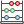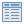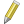# Field Calculator¶

TheField Calculator button in the attribute table allows to perform calculations on basis of existing attribute values or defined functions, e.g to calculate length or area of geometry features. The results can be written to a new attribute column or it can be used to update values in an already existing column.

You have to bring the vector layer in editing mode, before you can click on the field calculator icon to open the dialog (see figure_attributes_3). In the dialog you first have to select whether you want to only update selected features, create a new attribute field where the results of the calculation will be added or update an existing field.

Figure Attributes 3:

If you choose to add a new field, you need to enter a field name, a field type (integer, real or string), the total field width, and the field precision (see figure_attributes_3). For example, if you choose a field width of 10 and a field precision of 3 it means you have 6 signs before the dot, then the dot and another 3 signs for the precision.

The Function List contains functions as well as fields and values. View the help function in the Selected Function Help. In Expression you see the calculation expressions you create with the Function List. The most commonly used operators, see Operators.

In the Function List, click on Fields and Values to view all attributes of the attribute table to be searched. To add an attribute to the Field calculator Expression field, double click its name in the Fields and Values list. Generally you can use the various fields, values and functions to construct the calculation expression or you can just type it into the box. To display the values ​​of a field, you just right click on the appropriate field. You can choose between Load top 10 unique values and Load all unique values. On the right side opens the Field Values list with the unique values. To add a value to the Field calculator Expression box, double click its name in the Field Values list.

The Operators, Math, Conversions, String, Geometry and Record groups provides several functions. In Operators you find mathematical operators. Find Math for mathematical functions. The Conversions group contains functions that convert one data type to another. The String group provides functions for data strings. In the Geometry group you find functions for geometry objects. With Record group functions you can add a numeration to your data set. To add a function to the Field calculator Expression box, click on the > and then doubleclick the function.

A short example illustrates how the field calculator works. We want to calculate the length of the railroads layer from the QGIS sample dataset:

1. Load the Shapefile railroads.shp in QGIS and pressOpen Attribute Table.
2. Click onToggle editing mode and open theField Calculator dialog.
3. Select theCreate a new field checkbox to safe the calculations into a new field.
4. Add length as Output field name, real as Output field type and define Output field width 10 and a Precision of 3.
5. Now click on function length in the Geometry group to add it as \$length into the Field calculator expression box and click [Ok].
6. You can now find a new column length in the attribute table.

The available functions are listed below.

```column name "column name"            value of the field column name
'string'                             a string value
NULL                                 null value
a IS NULL                            a has no value
a IS NOT NULL                        a has a value
a IN (value[,value])                 a is below the values listed
a NOT IN (value[,value])             a is not below the values listed
a OR b                               a or *b* is true
a AND b                              a and *b* is true
NOT a                                inverted truth value of a
sqrt(a)                              square root of a
sin(a)                               sinus of a
cos(a)                               cosinus of b
tan(a)                               tangens of a
asin(a)                              arcussinus of a
acos(a)                              arcuscosinus of a
atan(a)                              arcustangens of a
to int(a)                            convert string a to integer
to real(a)                           convert string a to real
to string(a)                         convert number a to string
lower(a)                             convert string a to lower case
upper(a)                             convert string a to upper case
length(a)                            length of string a
atan2(y,x)                           arcustangens of y/x using the signs of the two
arguments to determine the quadrant of the result
replace(*a*, replacethis, withthat)  replace this with that in string a
regexp_replace(a,this,that)          replace the regular expression this with that
substr(*a*,from,len)                 len characters of string *a* starting from from
(first character index is 1)
a || b                               concatenate strings a and b
\$rownum                              number current row
\$area                                area of polygon
\$perimeter                           perimeter of polygon
\$length                              length of line
\$id                                  feature id
\$x                                   x coordinate of point
\$y                                   y coordinate of point
xat(n)                               X coordinate of the point of an n-th line (indeces
start at 0; negative values refer to the line end)
yat(n)                               y coordinate of the point of an n-th line (indeces
start at 0; negative values refer to the line end)
a = b                                a and b are equal
a != b and a <> b                    a and b are not equal
a >= b                               a is larger than or equal to b
a <= b                               a is less than or equal to b
a > b                                a is larger than b
a < b                                a is smaller than b
a ~ b                                a matches the regular expression b
a LIKE b                             a equals b
a ILIKE b                            a equals b (without regard to case-sensitive)
a |wedge| b                          a raised to the power of b
a * b                                a multiplied by b
a / b                                a divided by b
a + b                                a plus b
a - b                                a minus b
+ a                                  positive sign
- a                                  negative value of a```

The field calculator Function list with the Selected Function Help , Operators and Expression menu are also available through the rule-based rendering in the Style tab of the Layer properties and the expression based labelingin theLabeling core application.